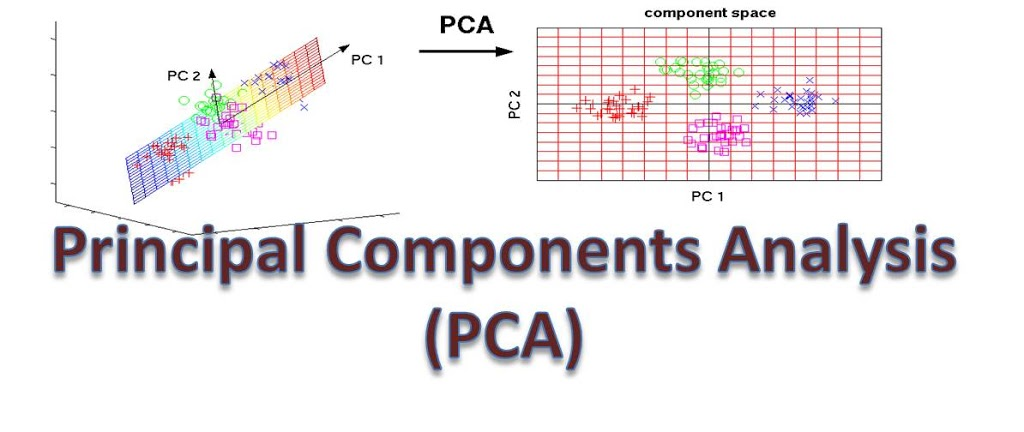# What is Principal Component Analysis (PCA) – A Simple TutorialIn this simple tutorial, I would explain the concept of Principal Components Analysis (PCA) in Machine Learning. I would try to be as simple and clear as possible.
The we would use Python in Tutorial 2 to actually do some of the hands-on, performing principal components analysis.

What is Principal Components Analysis?
Principal Components Analysis is an unsupervised learning class of statistical techniques used to explain data in high dimension using smaller number of variables called the principal components.
In PCA, we compute the principal component and used the to explain the data.

How PCA Work?
Assuming we have a set X made up of n measurements each represented by a set of p features, X1, X2, … , Xp. If we want to plot this data in a 2-dimensional plane, we can plot n measurements using two features at a time. If the number of features are more than 3 or four then plotting this in two dimension will be a challenge as the number of plots would be p(p-1)/2 which would be hard to plot.
We would like to visualize this data in two dimension without losing information contained in the data. This is what PCA allows us to do.

How to Computer Principal Components?
Given a dataset X of dimension n x p, how do we compute the first principal components?
To do this we look for linear combination of the feature values of the form:

that has the largest sample variance subject to the constraint that:

This means that  the first principal component loading vector solves the optimization problem such that we need to maximize the objective function subject to some constraint.
The objective function is given by:

And this is subject to the constraint:

The objective function (function to maximize) can be rewritten as:

Since this also holds:

Therefore the average of z11,…, zn1 will also be zero. Therefor the objective function that is being maximized is simply the sample variance of the n values of zi1.
z11, z2,…,zn1 are referred to as the scores of the first principal component.

How then do we maximize the given objective function?
We do this by performing eigen decomposition of the covariance matrix. Details of how to perform eigen decomposition is explained here.

Explaining the Principal Components
The loading vector Ф1 with elements Ф11, Ф21,…,Фp1  defines a direction in the feature space along which there is maximum variance in the data.
Thus, if we are to project the n data points x1, x2,…, xn onto this direction, then projected values are the actual principal component scores z11, z21, …, zn1.

After the first principal components, Z1 of the features has been determined, then the second principal component is the linear combination of X1, ,X2,… Xp that has the highest variance out of all the linear combinations that are uncorrelated with Z1. The second principal component scores z12, z22,…,zn2 take the form

where Ф2 is the second principal component loading vector, with elements Ф11, Ф12, … ,Фp2 . It turns out that constraining Z2 to be uncorrelated with Z1 is the same as constraining the direction of Ф2 to be orthogonal to the direction of  Ф1
We would now take an example to see how PCA works.